# How to Calculate Weighted Averages in Looker Studio

By Jack Zagorski | 5 min read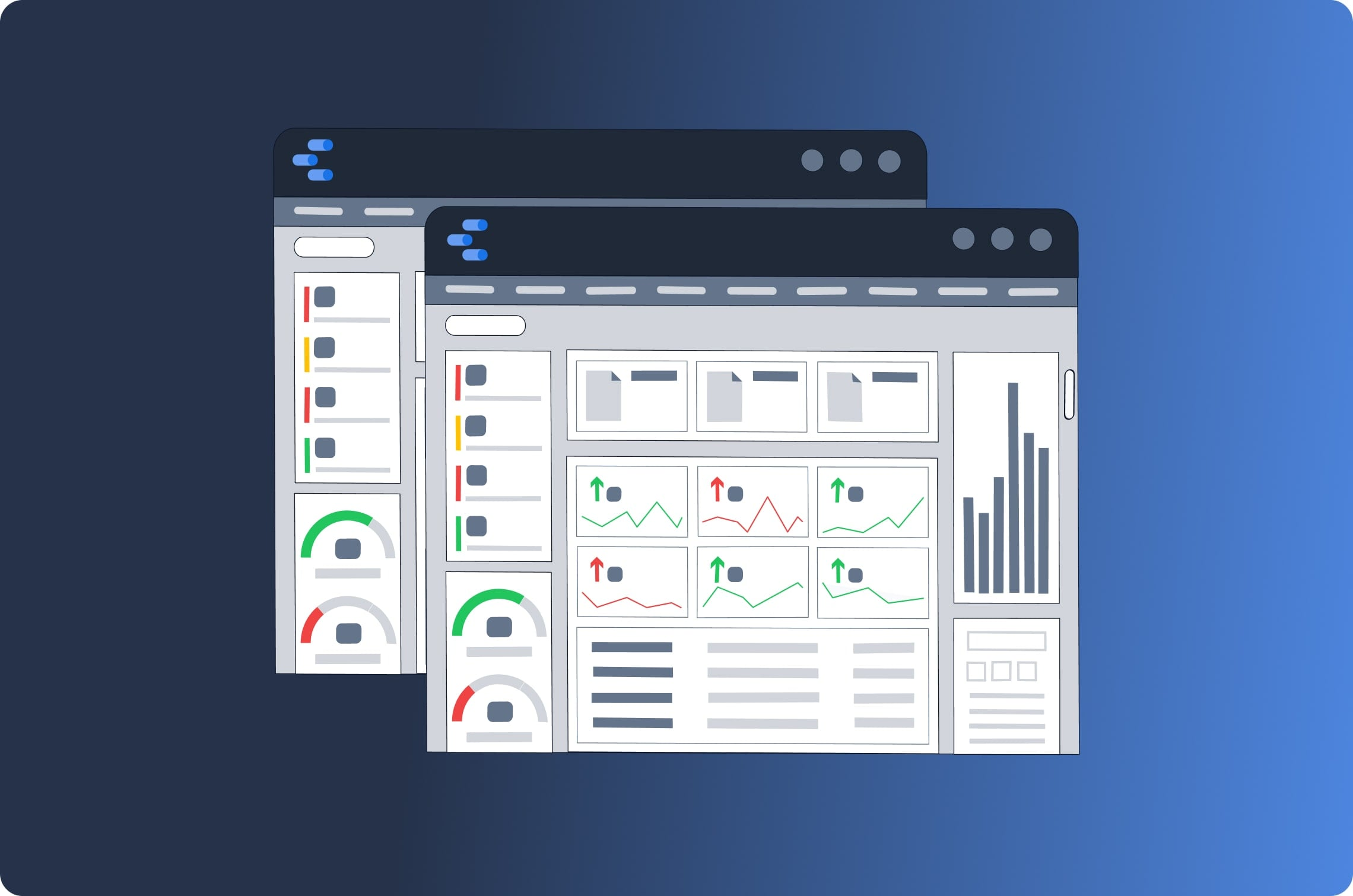If you use Looker Studio to track total calculated metrics, i.e. rates and averages across campaigns and time intervals, you will need to automate the calculation of weighted averages in Looker Studio to ensure that your numbers there match the numbers in your source data.

Calculated metrics include click-through rate (CTR), conversion rate (CR), cost per click (CPC), cost per mile/thousand (CPM), return on ad spend (ROAS), customer acquisition cost (CAC), and customer lifetime value (LTV).

Total calculated metrics cannot be aggregated over time intervals or campaigns. Nor can they be averaged simply. To get a truly accurate number, we have to calculate the weighted average.

## What Is Weighted Average?

Weighted average takes into account the varying degrees of importance (or weight) of numbers in a dataset.

For example, if you show an ad to 2 people and 1 of them converts, your conversion rate for this campaign would be 50%. If you show an ad to 100 people and 30 of them convert, your conversion rate would be 30%.

The simple average of these two conversion rates is of course 40%, but this is absolutely not the total conversion rate across campaigns because the conversion rate of 30% from the bigger campaign is much more important than the conversion rate of 50% from the smaller campaign.

The weighted average of these two campaigns, when rounded to the second decimal place, would actually still be 30%—30.4% to be exact!

## Looker Studio and Total Calculated Metrics

The best way to calculate total rates and averages in Looker Studio is to extract only the data that you need to calculate them (but not the rate and average data itself), then automate the calculations yourself in a calculated field.

The reasons for this are several:

1. Rate and average data is measured differently by different tools.
2. The extraction settings in your ETL tool can affect how Looker Studio automatically calculates rates and averages.
3. None of the settings in Looker Studio’s default summary row can calculate total rates and averages.

So, save yourself the trouble and don’t export native data for CTR, CR, CPC, or any other rate or average to your Looker Studio dashboard.

## Creating a Calculated Field for Weighted Average

To illustrate how to automate weighted average calculations, we will calculate the total CTR for one of our Facebook Ads campaigns over a 7-day period using clicks and impressions.

The basic formula for CTR is:

clicks / impressions = CTR

This formula will give us accurate numbers for row-level data (i.e. the data for each individual campaign or time interval; or in this case, for each day). But to get the weighted average for total CTR, we have to use the following formula:

sum (clicks) / sum (impressions) = total CTR

So, in Looker Studio’s right side panel, click Add a field.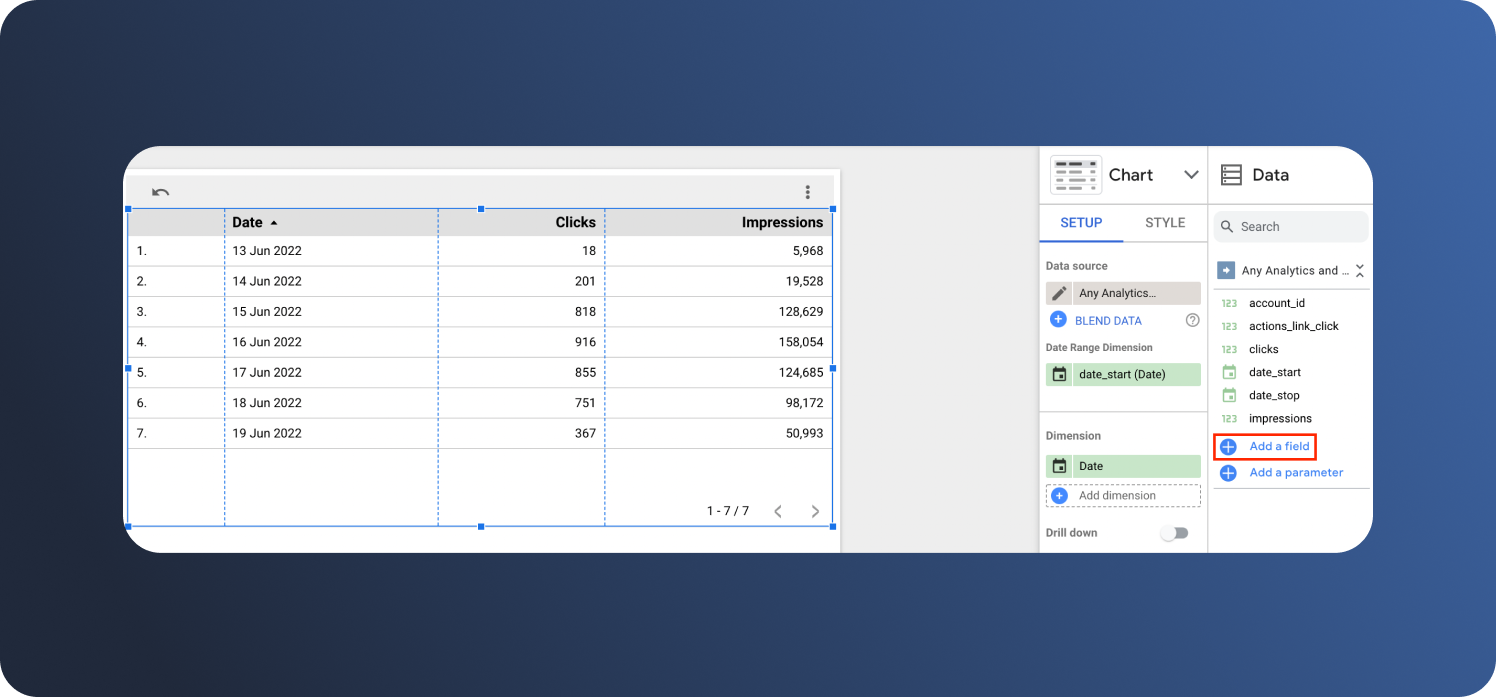Then, in the Formula field, type in SUM(clicks)/SUM(impressions). Use the automatic prompts to do this faster. Next, name the field and click save.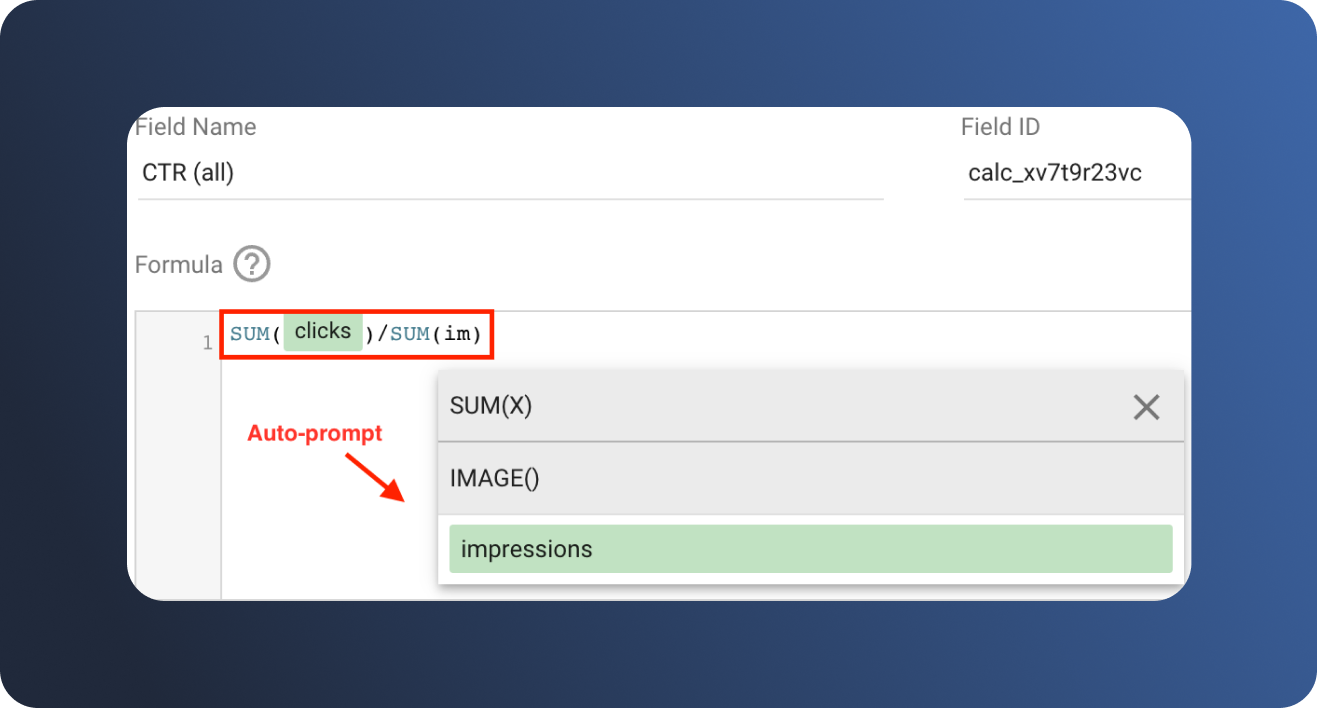Drag the new field, which in this example is called CTR (all), into the metric section. Then click AUT and modify the field to display as a percentage.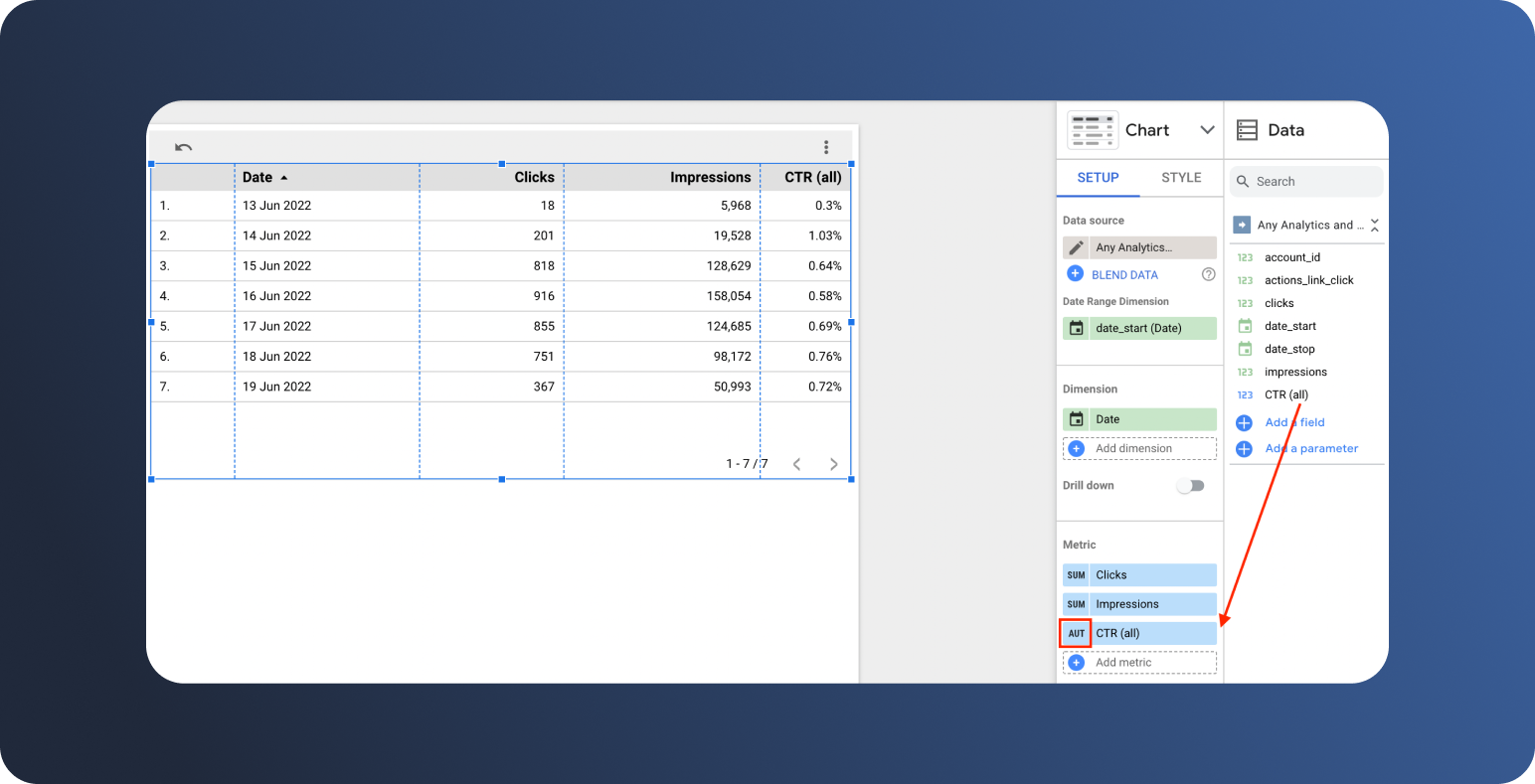Finally, check the Summary row box in the right side panel.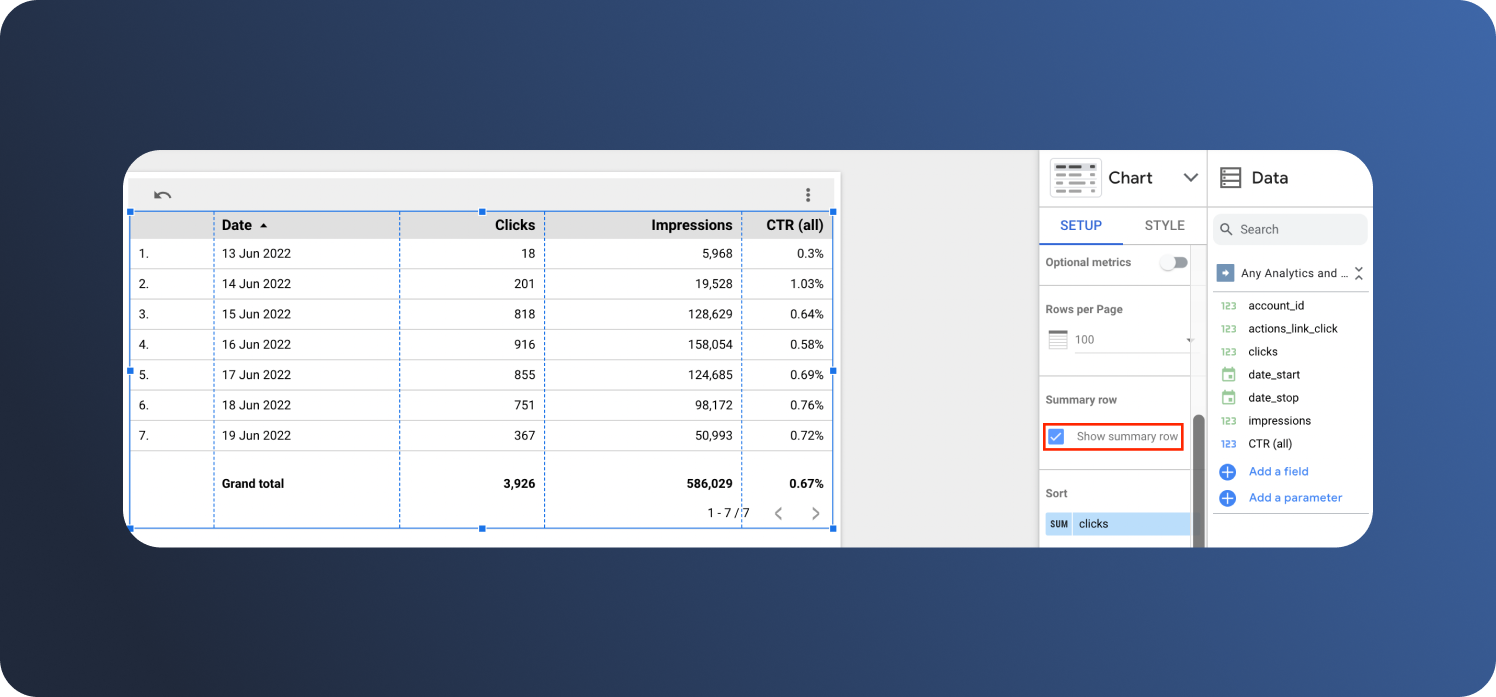Voila! You should now have accurate CTRs for all rows (simple averages) and an accurate total CTR for the summary row (a weighted average).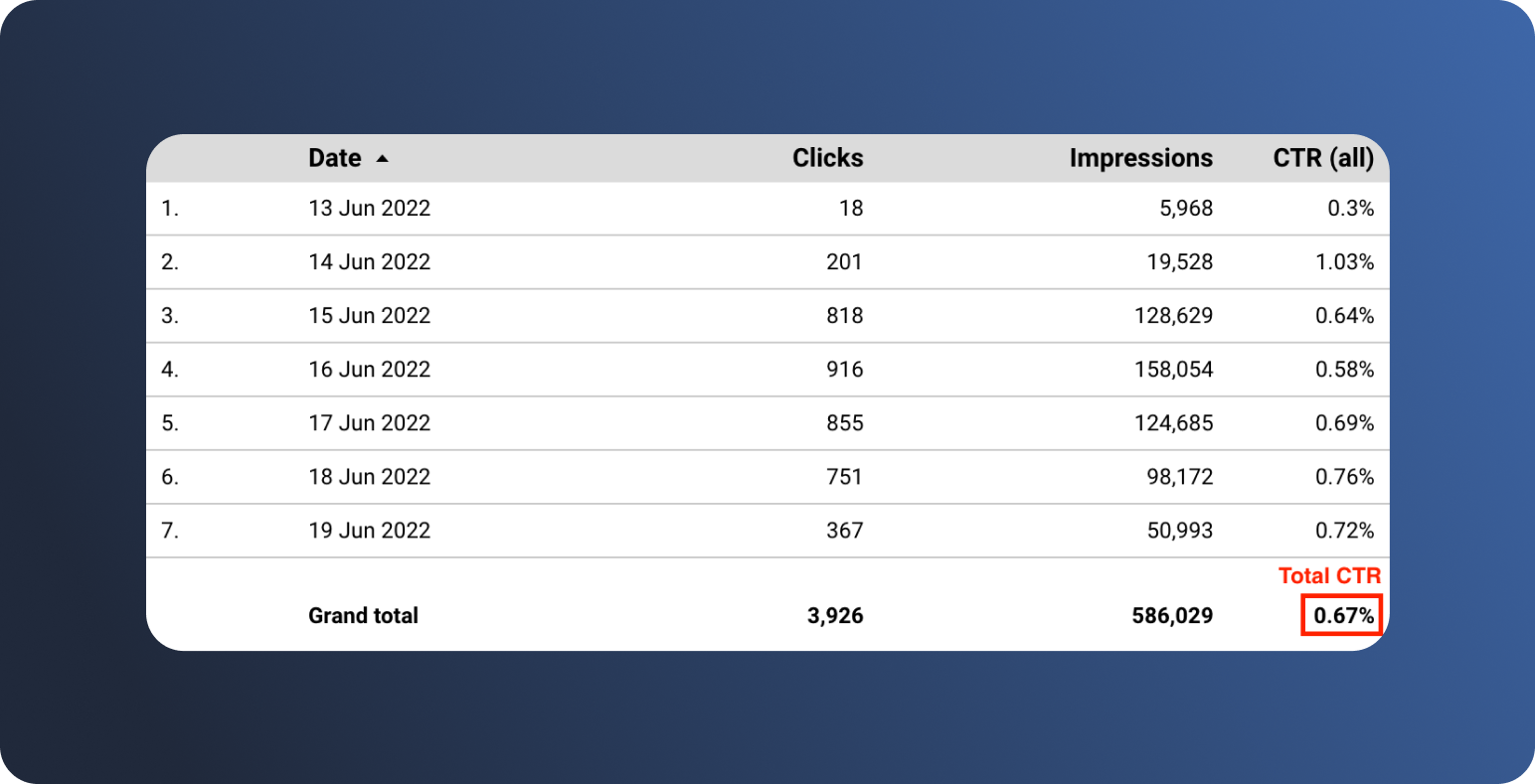The same type of summation formula would be used to get weighted averages for other total calculated metrics.

For example, the formula for total ROAS would be:

sum (revenue generated by ads) / sum (cost of ads) = total ROAS

Or the formula for total CPC would be:

sum (total advertising cost) / sum (total number of clicks) = total CPC

And so on…

Automating the calculation of weighted averages will do much ensure that the numbers in your Looker Studio dashboard line up with your source data.

Plus, some ETL tools charge according to the volume of data you extract, so if you’re using one of those, you’ll save money by extracting only the data you need to make your calculations, and then automating the calculations on your own!

 Extract any volume of data per source with Dataddo Dataddo's pricing plans are built around the number of sources you use, so you can extract as much data as you need and you'll never get a surprise bill at the end of the month.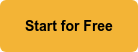Category: Tools